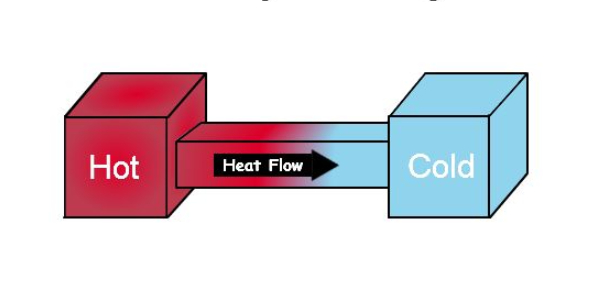11 Questions | Attempts: 1188
ShareSettingsThis quiz is to help students prepare for the Thermochemistry Exam

• 1.
Which of the following statements are correct?
• A.

ΔE is positive if work is done by the system and heat flows from the system

• B.

ΔE is only positive if q is positive.

• C.

ΔV is positive when work done by the system

• D.

ΔV is positive only when q is negative

• E.

W is negative when ΔV is positive

• 2.
The system absorbs 72 J of heat while 35 J of work is done on it.  What is the change in internal energy of the system.
• A.

107 J

• B.

37 J

• C.

-37 J

• D.

-107 J

• E.

None of these.

• 3.
You have two pieces of different metals but they have the same mass.  If you subjected both of them to the same amount of energy, what would happen?
• A.

Their temperatures would both increase at the same rate.

• B.

The metal with the lower heat capacity would undergo a lower temperature change.

• C.

The metal with the higher heat capacity would undergo a higher temperature change.

• D.

The metal with the lower heat capacity would undergo a higher temperature change.

• E.

None of these.

• 4.
A 150.0 g sample of metal at 75.0 C is added to 150.0 g of H2O at 15.0 C.  The temperature of the water rises to 18.3 C.  Calculate the heat capacity of the metal, assuming that all the heat lost by the metal is gained by the water.  The heat capacity of water is 4.18 J/g C
• A.

0.22 J/ g C

• B.

0.25 J/ g C

• C.

0.23 J/ g C

• D.

0.48 J/ g C

• E.

0.43 J/ g C

• 5.
How much heat would be required to heat 150. g of water at 25.0 C to boiling point? The specific heat of water is 4.18 J/g C
• A.

4.70 kJ

• B.

47.0 kJ

• C.

470 J

• D.

1.57 kJ

• E.

15.7 kJ

• 6.
If the heat of combustion of H2 = -572 kJ.  What is the change in enthalpy of the reaction if you have 46.1 g of hydrogen gas?
• A.

1.31 x 10^4 kJ

• B.

-1.31 x 10^4 kJ

• C.

2.61 x 10^4 kJ

• D.

-2.61 x 10^4 kJ

• E.

None of these.

• 7.
The combustion of methane (CH4) releases 891 kJ of energy.  The heat of formations of CO2 = -393 kJ/mol and H2O(l) = -286 kJ/mol.  What is the heat of formation of methane?
• A.

-1570 kJ

• B.

-1865

• C.

-74 kJ

• D.

74 kJ

• E.

212 kJ

• 8.
Which of the following are not a form of fossil fuels?
• A.

Coal

• B.

Natural gas

• C.

Diesel fuel

• D.

Propane

• E.

CO2

• 9.
Which of the following is not considered an alternate energy source?
• A.

Hydrogen gas

• B.

Ethanol

• C.

Gasohol

• D.

Biodiesel

• E.

Methanol

• F.

All of these are considered alternate energy sources.

• 10.
What does the First Law of Thermodynamics mean and how does the system change its internal energy?
• A.

The First Law of Thermodynamics states that energy is conserved and the change in internal energy of the system is always zero.

• B.

The First Law of Thermodynamics states that energy not created nor destroyed and the internal energy of the system is in constant change.

• C.

The First Law of Thermodynamics states that energy in the universe is conserved and the internal energy of the system changes due to work and heat flow to and from the surroundings.

• D.

The First Law of Thermodynamics states that energy is not created nor destroyed but the internal energy of the system is changing because heat is always flowing.

• E.

The First Law of Thermodynamics states that energy in the universe is conserved and the internal energy of the system changes due to work and heat constantly flowing to the surroundings.

• 11.
When calculating the heat of a reaction, you have to know the heat of formations of the products and reactants. Some of these substances always have a heat of formation of zero.  Why is this?
• A.

Elements standing alone always have a heat of formation of zero.

• B.

Compounds, such as water, in their standard state are always zero.

• C.

Gases are always zero because they are freely formed in the atmosphere.

• D.

Elements standing alone in their standard state are always zero.

• E.

Water is the only one that is zero.

## Related TopicsBack to top
×

Wait!
Here's an interesting quiz for you.